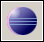# JAVA – Parsing given string to be used by java.awt.robotJava Robot can be very usefull tool emulating e.g. pressing a key on your keyboard. Sometimes this can be difficult especially for non-standard characters like ‘asterisk’. Therefore I developed following piece of code which translates given string into separated chars, converts them to ASCII and Java Robot types them using ALT+ASCII_KEY

```// The purpose is to translate given string to sparated characters
// which are typed one by one using their corresponding ASCII value
// This value is then translated to KeyCodes
private void TypeString(String data){
// Example data: data = "Hello World"
// First loop: c = 'H'
for (char c : data.toCharArray()){
//  ASCII code for 'H': charcode_int = 72
int charcode_int = (int)c;

//  charcode = "72"
String charcode = Integer.toString(charcode_int);
robot.keyPress(KeyEvent.VK_ALT);

//  First loop: ascii_c = '7'
for (char ascii_c : charcode.toCharArray()){
//    ascii_n = 103 (= VK_NUMPAD7)
int ascii_n = Integer.parseInt(String.valueOf(ascii_c)) + 96;
robot.keyPress(ascii_n);
robot.keyRelease(ascii_n);
}

robot.keyRelease(KeyEvent.VK_ALT);
}
}```